Daily Practice Problems
Class 6 Maths
FractionsQuestion 1.

Represent the following fractions on number line.

(a)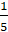(b)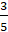Question 2:

Write all the natural numbers from 1 to 20. What fraction of them are prime numbers?

Question 3:

Convert the following improper fractions into mixed fraction.

(a)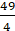(b)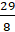Question 4:

Write the following fractions in ascending order: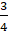,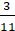,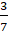,,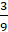Question 5:

Compare the following fractions: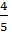and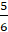Question 6:

ADD: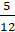,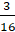and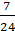Question 7:

Simran painted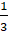of the wall space in her room. Her brother Rahul helped and painted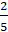of the wall space. How much did they paint together? What part of the whole space is left unpainted?

Question 8:

Write the fraction representing the shaded portion.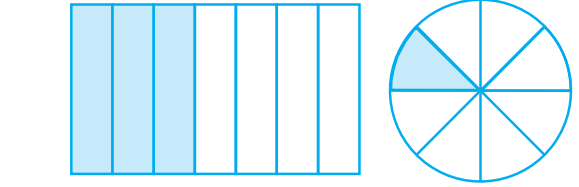(i)                                 (ii)

Question 9:

State true or false:

(a) In, 3 is the part of whole.

(b) On a number line,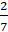is to the right of zero.

(c)is smaller than.

(d)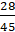andrepresent equivalent fractions.

Question 10:

Find the missing entries in the tables: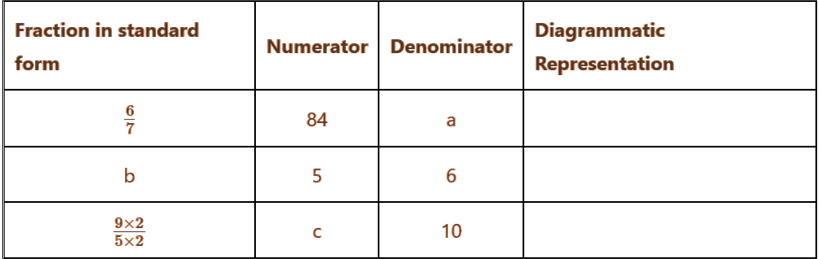Question 10:

Show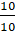on the number line.

Question 12:

Match the following: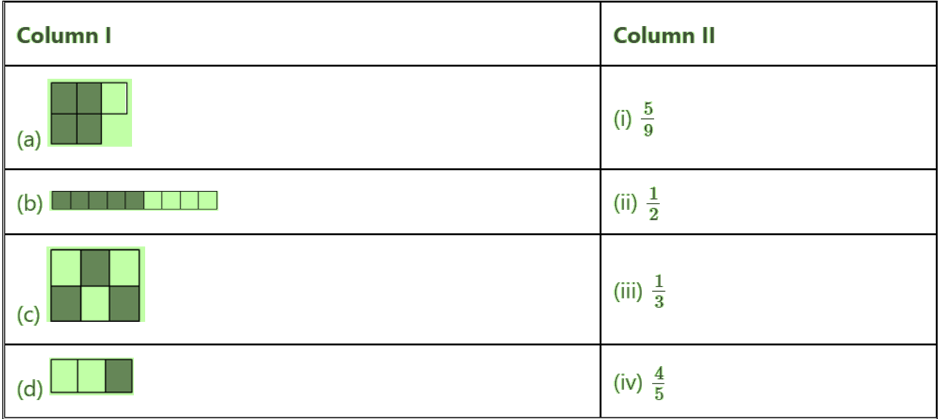Question 13:

There is a large box of 36 small square boxes.

(a)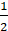of it is _____.

(b)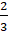of it is _____.

(c) If I make a bench of 20 small boxes, the fraction becomes _____.

(d) _____ boxes are required if fraction is 56.

Question 14:

Subtract 2from 4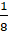.

Question 15:

Find the equivalent fraction ofhaving

(i) denominator 30

(ii) numerator 24.

Question 16:

Identify the proper and improper fractions: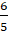,,,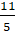.

Question 17:

Identify the like fractions from the following:,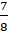,,,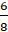Question 18:

Write any

(a) three proper and three improper fractions with denominator 9.

(b) two proper and two improper fractions with numerator 7.

Question 19:

Simplify:–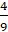+Question 20:

What fraction of an hour is 45 minutes?

*********